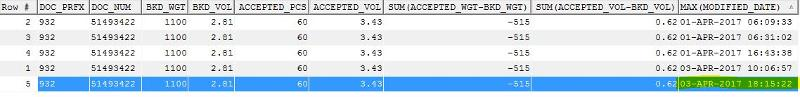SQL query to select row with MAX dateJason Palmer used Ask the Experts™
on
I'm trying to return the row with the MAX date but the MAX funciton doesn't appear to be working. Below is the code I'm trying to run along with the output: Any help you can provide will be very much appreciated.

select doc_prfx, doc_num, BKD_WGT, BKD_VOL, ACCEPTED_PCS, ACCEPTED_VOL, sum (ACCEPTED_WGT - BKD_WGT), sum (ACCEPTED_VOL - BKD_VOL), MAX(modified_date)

from

(Select distinct
c.car_id,
b.doc_prfx, b.doc_num,

f.wt_bfr_awb as BKD_WGT, f.vol_bfr_awb as BKD_VOL,

g.num_pcs as ACCEPTED_PCS,
g.ttl_wt as ACCEPTED_WGT, g.vol as ACCEPTED_VOL,

to_char(f.modified_date,  'DD-MON-YYYY HH24:MI:SS') as modified_date

from sbh_res_itnry a, sbh_car b, sbh_res c, sbh_res_cust d, fwb_res_data_aud e, sbh_res_aud f, sbh_AWB g
where c.car_id = b.car_id (+)
and c.car_id = a.car_id
and c.car_id = d.car_id
and b.car_id = e.car_id (+)
and c.car_id = f.car_id
and c.car_id = g.car_id
and a.aln_code = 'VS'
and a.flt_num = '0003'
and trunc (a.dep_date) = '04-APR-2017'
--and e.rmk_typ = 'BKL' --in (null,'BKL')
AND a.cncl_ind = 'N'
and d.cust_typ = 'AGT'
and b.cncl_ind = 'N'
and c.enq_ind = 'N'
and f.wt_bfr_awb is not null
and b.doc_prfx = '932'
and b.doc_num in ('51493422')
)
group by

doc_prfx, doc_num, BKD_WGT, BKD_VOL, ACCEPTED_PCS, ACCEPTED_VOL,modified_date
Comment
Watch Question

Do more withEXPERT OFFICE® is a registered trademark of EXPERTS EXCHANGE®
Senior Developer

Commented:
To filter by that, you need a subquery. The MAX() aggregate itself does only a calculation. The normal approach is:

SELECT *
FROM yourQuery
WHERE dateColumn = (
SELECT MAX(dateColumn)
FROM yourQuery
);

Depending on the problem, the subquery must be correlated.
All sorted, thank you
Senior Oracle DBA
Commented:
You don't need the subquery to get the max.  You can avoid hitting the table twice like that by using windowed aggregates.

A simple example would be:
SELECT doc_num,
modified_date
FROM   (
SELECT   doc_num,
modified_date,
row_number() over (paratition BY doc_num ORDER BY modified_date DESC) rn
FROM     you_tab)
WHERE  rn = 1;
Senior Developer

Commented:
Caveat: Test always both approaches. Cause ROW_NUMBER() is not necessarily always better.
Senior Oracle DBA
Commented:
In most, but definitely not all, cases ROW_NUMBER will perform better as it only has to hit the base table once.
Achieve the outcome i needed as shown below

select distinct doc_pfx, doc_num, AWB_BKD_WGT, AWB_BKD_VOL,PART_SHPMT_IND,
FLT_BKD_WGT, FLT_BKD_VOL,
ACCEPTED_WGT, ACCEPTED_VOL,

WGT_VAR, VOL_VAR
modified_date

from

(Select
--c.car_id,
b.doc_prfx as DOC_PFX, b.doc_num,

a.bkd_wt as FLT_BKD_WGT, a.bkd_vol as FLT_BKD_VOL,
f.wt_bfr_awb as AWB_BKD_WGT, f.vol_bfr_awb as AWB_BKD_VOL,
a.part_shpmnt_ind as PART_SHPMT_IND,

g.num_pcs as ACCEPTED_PCS,
g.ttl_wt as ACCEPTED_WGT, g.vol as ACCEPTED_VOL,
g.ttl_wt - f.wt_bfr_awb as WGT_VAR,
g.vol - f.vol_bfr_awb  as VOL_VAR,

MAX(to_char(f.modified_date,  'DD-MON-YYYY HH24:MI:SS')) as modified_date

from sbh_res_itnry a, sbh_car b, sbh_res c, sbh_res_cust d, sbh_res f, sbh_AWB g
where c.car_id = b.car_id (+)
and c.car_id = a.car_id
and c.car_id = d.car_id

and c.car_id = f.car_id
and c.car_id = g.car_id
and a.aln_code = 'VS'
and a.flt_num = '0003'
and trunc (a.dep_date) = '04-APR-2017'
AND a.cncl_ind = 'N'
and d.cust_typ = 'AGT'
and b.cncl_ind = 'N'
--and c.enq_ind = 'N'
--and f.wt_bfr_awb is not null
--and b.doc_prfx = '932'
--and b.doc_num in ('51493422','51669634')

group by
b.doc_prfx, b.doc_num,
f.wt_bfr_awb, f.vol_bfr_awb,
g.num_pcs,
g.ttl_wt, g.vol,a.part_shpmnt_ind,
a.bkd_wt, a.bkd_vol
)

Commented:
thanks for you help guys

Do more withSubmit tech questions to Ask the Experts™ at any time to receive solutions, advice, and new ideas from leading industry professionals.